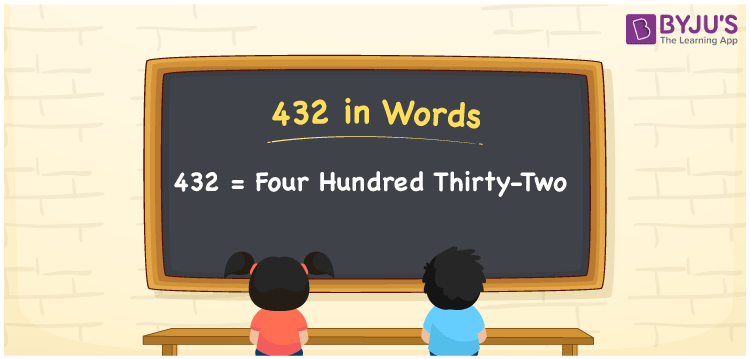# 432 in Words

432 in words is written as Four Hundred Thirty-two. The name of number 432 in English is “Four Hundred Thirty-two”. The word Four Hundred Thirty-two is used in many real-life situations. For example, there are Four Hundred Thirty-two students in a classroom. Thus, it is a counting number. Also, to represent the currency equal to 432, we can write it in words as Rupees Four Hundred Thirty-two or Four Hundred Thirty-two rupees. Therefore, it is necessary to learn the numbers in words, for the ease of understanding and expressing them.

 432 in words Four Hundred Thirty-two Four Hundred Thirty-two in Numbers 432

## 432 in English Words## How to Write 432 in Words?

If we know the place value of digits of 432, then we can easily express it in words. The place value is basically the position of a digit in a number. 432 is a three-digit number, therefore, we can specify the position of each digit of 432 in a place value chart. In the Indian numbering system, the order of place value of digits from right to left is given by:

 Hundreds Tens Ones 4 3 2

We can write it in expanded form as:

4 × Hundred + 3 × Ten + 2 × One

= 4 × 100 + 3 × 10 + 2 × 1

= 400 + 30 + 2

= 432

= Four Hundred Thirty-two

Therefore, 432 in words is written as eight hundred thirties.

432 is a natural number that precedes 433 and succeeds 431.

432 in words – Four Hundred Thirty-two

Is 432 an odd number? – No

Is 432 an even number? – Yes

Is 432 a perfect square number? – No

Is 432 a perfect cube number? – No

Is 432 a prime number? – No

Is 432 a composite number? – Yes

## Frequently Asked Questions on 432 in Words

Q1

### How to write 432 in words?

432 in English is written as “Four Hundred Thirty-two”.
Q2

### Is 432 an odd number or even number?

432 is an even number because it is wholly divisible by 2.
Q3

### Write Four Hundred Thirty-two in numbers.

Four Hundred Thirty-two in numbers is 432.